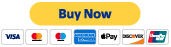#### Part 1

Discover key mathematical concepts such as decomposing numbers, rounding to the nearest 10, 100, or 1,000, comparing and ordering numbers, and equivalent fractions on the number line.

TEKS 3.2a, c-d, 3.2f

#### Part 5

Examine geometry and measurement concepts like describing 3D shapes using formal language, quadrilaterals and their various classifications, and the area of rectangles.

TEKS 3.6a-c

#### Part 2

Explore the first part of operations by looking at how to compare fractions using models, even and odd numbers, more fractions on a number line, and addition and subtraction.

TEKS 3.3h, 3.4a,i, 3.7a

#### Part 6

Take a look at geometry, measurement, and data analysis concepts such as finding the perimeter, calculating intervals of time, and frequency table, dot plots, pictographs, and bar graphs.

TEKS 3.7b-c, 3.8a-b

#### Part 3

Take a look at more essential operations, including 2 by 1 multiplication, division as equal shares/groups, multiplication and division facts, and representing addition and subtraction.

TEKS 3.4f-h, k, 3.5a

#### Part 4

Uncover operations and algebraic expressions, such as representing multiplication and division, an introduction to long division, input-output tables, and counting a collection of coins and bills.

TEKS 3.4c, j, 3.5b, eTake the 6 hour CPE course (\$20)

Shortly after payment (usually less than 1 hour), you will receive an email with the password to the quizzes.

## Quizzes

Click the button below to access the quizzes for each part. Credit will not be earned until the CPE course has been purchased and all quizzes have been completed.

###### Contact me!

Tel: 817-681-8854

aarondaffern@gmail.com

© 2020 by Aaron Daffern Consulting